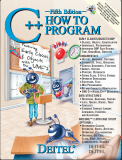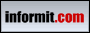Back to www.deitel.comISBN: 0-13-185757-6 © 2005 pages: 1500 Buy the Book!C++ allows programmers to specify how operators work with objects of new class types--a concept known as operator overloading. One example of an overloaded operator built into C++ is <<, which is used both as the stream insertion operator and as the bitwise left-shift operator. Similarly, >> is used as both the stream extraction operator and as the bitwise right-shift operator.

This tutorial discusses an Array class that overloads several operators. Our Array class provides enhanced functionality over traditional C++ arrays, such as assigning and comparing Array objects, and checking array indices to ensure that we do not access elements outside the bounds of the underlying C++ array. In addition, this tutorial introduces a copy constructor for initializing a new Array object with the contents of an existing Array object. This tutorial is intended for students and professionals who are familiar with basic array, pointer and class concepts in C++.

[Note: This tutorial is an excerpt (Section 11.8) of Chapter 11, Operator Overloading, from our textbook C++ How to Program, 5/e. These tutorials may refer to other chapters or sections of the book that are not included here. Permission Information: Deitel, Harvey M. and Paul J., C++ HOW TO PROGRAM, ©2005, pp.582-593. Electronically reproduced by permission of Pearson Education, Inc., Upper Saddle River, New Jersey.]

11.8 Case Study: `Array` Class (Continued)

 ``` 1 // Fig 11.7: Array.cpp 2 // Member-function definitions for class Array 3 #include 4 using std::cerr; 5 using std::cout; 6 using std::cin; 7 using std::endl; 8 9 #include 10 using std::setw; 11 12 #include // exit function prototype 13 using std::exit; 14 15 #include "Array.h" // Array class definition 16 17 // default constructor for class Array (default size 10) 18 Array::Array( int arraySize ) 19 { 20 size = ( arraySize > 0 ? arraySize : 10 ); // validate arraySize 21 ptr = new int[ size ]; // create space for pointer-based array 22 23 for ( int i = 0; i < size; i++ ) 24 ptr[ i ] = 0; // set pointer-based array element 25 } // end Array default constructor 26 27 // copy constructor for class Array; 28 // must receive a reference to prevent infinite recursion 29 Array::Array( const Array &arrayToCopy ) 30 : size( arrayToCopy.size ) 31 { 32 ptr = new int[ size ]; // create space for pointer-based array 33 34 for ( int i = 0; i < size; i++ ) 35 ptr[ i ] = arrayToCopy.ptr[ i ]; // copy into object 36 } // end Array copy constructor 37 38 // destructor for class Array 39 Array::~Array() 40 { 41 delete [] ptr; // release pointer-based array space 42 } // end destructor 43 44 // return number of elements of Array 45 int Array::getSize() const 46 { 47 return size; // number of elements in Array 48 } // end function getSize 49 50 // overloaded assignment operator; 51 // const return avoids: ( a1 = a2 ) = a3 52 const Array &Array::operator=( const Array &right ) 53 { 54 if ( &right != this ) // avoid self-assignment 55 { 56 // for Arrays of different sizes, deallocate original 57 // left-side array, then allocate new left-side array 58 if ( size != right.size ) 59 { 60 delete [] ptr; // release space 61 size = right.size; // resize this object 62 ptr = new int[ size ]; // create space for array copy 63 } // end inner if 64 65 for ( int i = 0; i < size; i++ ) 66 ptr[ i ] = right.ptr[ i ]; // copy array into object 67 } // end outer if 68 69 return *this; // enables x = y = z, for example 70 } // end function operator= 71 72 // determine if two Arrays are equal and 73 // return true, otherwise return false 74 bool Array::operator==( const Array &right ) const 75 { 76 if ( size != right.size ) 77 return false; // arrays of different number of elements 78 79 for ( int i = 0; i < size; i++ ) 80 if ( ptr[ i ] != right.ptr[ i ] ) 81 return false; // Array contents are not equal 82 83 return true; // Arrays are equal 84 } // end function operator== 85 86 // overloaded subscript operator for non-const Arrays; 87 // reference return creates a modifiable lvalue 88 int &Array::operator[]( int subscript ) 89 { 90 // check for subscript out-of-range error 91 if ( subscript < 0 || subscript >= size ) 92 { 93 cerr << "\nError: Subscript " << subscript 94 << " out of range" << endl; 95 exit( 1 ); // terminate program; subscript out of range 96 } // end if 97 98 return ptr[ subscript ]; // reference return 99 } // end function operator[] 100 101 // overloaded subscript operator for const Arrays 102 // const reference return creates an rvalue 103 int Array::operator[]( int subscript ) const 104 { 105 // check for subscript out-of-range error 106 if ( subscript < 0 || subscript >= size ) 107 { 108 cerr << "\nError: Subscript " << subscript 109 << " out of range" << endl; 110 exit( 1 ); // terminate program; subscript out of range 111 } // end if 112 113 return ptr[ subscript ]; // returns copy of this element 114 } // end function operator[] 115 116 // overloaded input operator for class Array; 117 // inputs values for entire Array 118 istream &operator>>( istream &input, Array &a ) 119 { 120 for ( int i = 0; i < a.size; i++ ) 121 input >> a.ptr[ i ]; 122 123 return input; // enables cin >> x >> y; 124 } // end function 125 126 // overloaded output operator for class Array 127 ostream &operator<<( ostream &output, const Array &a ) 128 { 129 int i; 130 131 // output private ptr-based array 132 for ( i = 0; i < a.size; i++ ) 133 { 134 output << setw( 12 ) << a.ptr[ i ]; 135 136 if ( ( i + 1 ) % 4 == 0 ) // 4 numbers per row of output 137 output << endl; 138 } // end for 139 140 if ( i % 4 != 0 ) // end last line of output 141 output << endl; 142 143 return output; // enables cout << x << y; 144 } // end function operator<< ```

Fig. 11.7 `Array` class member- and `friend`-function definitions.

Page 1 | 2 | 3 | 4 | 5 | 6 | 7 | 8 | 9 | 10
Tutorial Index